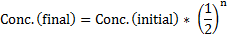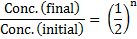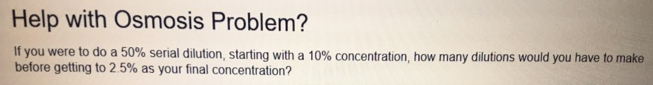# Problem: If you were to do a 50% serial dilution, starting with a 10% concentration, how many dilutions would you have to make before getting 2.5% as your final concentration?

###### FREE Expert Solution

Simply put, a serial 50% dilution is a series of 50% dilutions, each resulting in half the preceding concentration. The formula below can simply be followed:This can be rearranged to getThe number of dilutions, n, can be computed using natural logarithm.###### Problem Details

If you were to do a 50% serial dilution, starting with a 10% concentration, how many dilutions would you have to make before getting 2.5% as your final concentration?